Courses

# Power Systems - 1

## 10 Questions MCQ Test GATE Electrical Engineering (EE) 2022 Mock Test Series | Power Systems - 1

Description
This mock test of Power Systems - 1 for Electrical Engineering (EE) helps you for every Electrical Engineering (EE) entrance exam. This contains 10 Multiple Choice Questions for Electrical Engineering (EE) Power Systems - 1 (mcq) to study with solutions a complete question bank. The solved questions answers in this Power Systems - 1 quiz give you a good mix of easy questions and tough questions. Electrical Engineering (EE) students definitely take this Power Systems - 1 exercise for a better result in the exam. You can find other Power Systems - 1 extra questions, long questions & short questions for Electrical Engineering (EE) on EduRev as well by searching above.
QUESTION: 1

### The main objective of the governor system in power systems for all types of turbines is to control the:

Solution:

The main objective of the governor system in power systems for all types of turbines is to control the frequency. A governor protects the prime mover from over speed and keeps the prime mover speed at or near the desired revolutions per minute. When a prime mover drives an alternator supplying electrical power at a given frequency, a governor must be used to hold the prime mover at a speed that will yield this frequency.

*Answer can only contain numeric values
QUESTION: 2

### In a hydroelectric plant, the available head is 80 m for a discharge 12m3/sec, and overall efficiency of the PowerStation of 80%. The specific weight of water is 9.81 k/m2. Then the power developed is – (in MW)

Solution:

P = WQHη KW

= 9.81 × 12 × 80 × 0.8

= 7534.08 KW = 7.53 MW

*Answer can only contain numeric values
QUESTION: 3

### An industrial consumer has a daily load pattern of 2500 kW, 0.8 lag for 8 hours, 2000 kW, 0.6 lag for 8 hours and 1500 kW, unity power factor for 8 hours. What is the load factor?

Solution: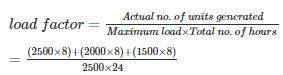= 48000/60000

= 0.8

QUESTION: 4

Consider a power system with three identical generators. The transmission losses are negligible. One generator(G1) has a speed governor which maintains its speed constant at the rated value, while the other generators (G2 and G3) have governors with a drop of 5%. If the load of the system is increased, then in steady state.

Solution:

Given power system with these identical generators G1 has Speed governor G2 and G3 has drop of 5% When load increased, in steady state generation of G1 is only increased while generation of G2 and G3 are unchanged.

QUESTION: 5

consider the two bus power system network with given loads as shown in the figure. All the value is shown in the figure in per unit. The reactive power supplied by generator G1 and G2 is QG1and QG2 respectively. The per unit values of QG1­, QG2 and line reactive power loss Qloss respectively areSolution:

Loss less line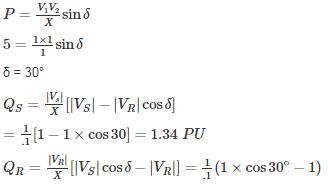= -1.34 PU

∴ QG1 = 5 + 1.34 = 6.24 PU

QL2 = QG2 + QR

QG2 = 10 – (-1.34) = 11.34 PU

Qloss = QS - QP = 1.34 – (-1.34) = 2.68

*Answer can only contain numeric values
QUESTION: 6

A generating station has the following data:

Installed capacity = 420 MW; capacity factor = 60%

Annual load factor = 70%.

The minimum reserve capacity of the station is – (in MW)

Solution: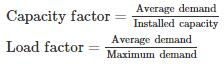By above two equations,
Maximum demad = Installed capacity x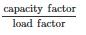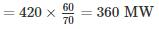Reserve capacity = Installed capacity − Maximum demand
= 420 – 360 = 60 MW

*Answer can only contain numeric values
QUESTION: 7

Determine the incremental cost of received power of the plant shown in the figure below, if the incremental cost of production is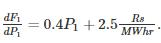________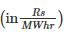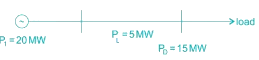Solution:

Penalty Factor = 20/15 = 4/3

Cost of received power =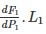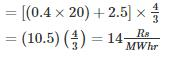QUESTION: 8

Assume that the fuel input in Btu per hour for units 1 and 2 are given by
F1 = (4P1 + 0.036P21 + 60)106
F2 = (3P2 + 0.02P22 + 100)106
The maximum and minimum loads on the units are 100 MW and 10 MW respectively. The minimum cost of a generation when the following load is supplied is _______ (in Rs.) Where the cost of fuel is Rs. 1 per million Btu. (Approximately)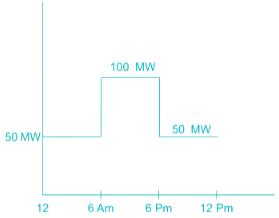Solution:

From the fuel input characteristics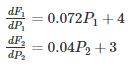When the load is 50 MW, for economic loading.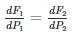⇒ 0.072P1 + 4 = 0.04 P2 + 3

⇒ 0.072P1 – 0.04P2 + 1 = 0

P1 + P2 = 50

⇒ P1 = 8.93 MW P2 = 41.07 MW.

Minimum load on P1 = 10 MW

⇒ (i) P1 = 10 MW, P2 = 40 MW (or)

(ii)  P1 = 0 MW, P2 = 50 MW

(i)  P1 = 10 MW, P2 = 40 MW

F1 = 103.6 million Btu / hr

F2 = 252 million Btu / hr

F1 + F2 = 355.6 million Btu / hr

(ii)  P1 = 0 MW, P2 = 50 MW

F1 = 60 million Btu / hr

F2 = 300 million Btu / hr

F1 + F2 = 360 million Btu / hr

So for P1 = 10 MW, P2 = 40 MW, the fuel cost is less.

When the load is 100 MW.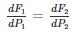⇒ 0.072 P− 0.04 P+ 1 = 0

⇒ P1 + P2 = 100

⇒ P1 = 26.79 P2 = 73.21

F1 = 193 million Btu / hr

F2 = 512.58 million Btu / hr

Now, total fuel cost

= (103.60 + 252 + 193 + 512.58) (12) (1)

= Rs. 12734.16

*Answer can only contain numeric values
QUESTION: 9

A steam power station spends Rs. 30 lakhs per annum for coal used in the station. The coal has a calorific value of 5000 kcal/kg and costs Rs. 300 per ton. If the station has thermal efficiency of 33% and electrical efficiency of 90%, find the average load on the station. (in kW)

Solution:

Overall efficiency, ηoverall = 0.33 × 0.9 = 0.297
Coal used/annum =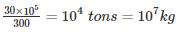Heat of combustion = coal used/annum × calorific value

= 107 × 5000 = 5 × 1010 kcal

Heat output = ηoverall × heat of combustion

= (0.297) × (5 × 1010) = 1485 × 107 kcal
Units generated per annum =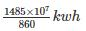Average load on station =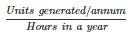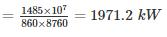QUESTION: 10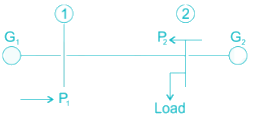In the above system shown, a transmission loss of 2.5 MW is incurred if 50 MW is transmitted from. Plant – 1 to the load LaGrange’s multiplier λ is Rs. 20 / MWH.

The incremental fuel cost is given.

IC1 = 0.01P+ 12Rs/MWH,IC2 = 0.04P2 + 16Rs/MWH
For optimal load scheduling.

The powers of plants 1 and plant 2 and losses are respectively.

Solution:

Loss PL= B11P21

Load Power PD = P1 + P2 − PL

For P1 = 50MW,PL = 2.5

So, 2.5 = B11 × (50)2

⇒ B11 = 0.001 MW−1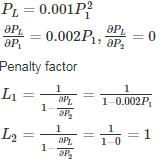For optimal load scheduling.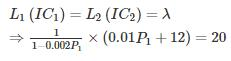And
1 × (0.04P2 + 16) = 20
P2 = 100MW
0.01P1 + 12 = 20(1 − 0.002P1)
P1 = 160MW
PL = 0.001 × (160)2 = 25.6MW
PP = 100 + 160 − 25.6 = 234.4MW